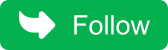## Monday, May 2, 2022

### Question 9 : Write a program to print all permutations of String in java?

A permutation is an arrangement of all or part of a set of objects, with regard to the order of the arrangement. For instance, the words ‘bat’ and ‘tab’ represents two distinct permutation (or arrangements) of a similar three-letter word.

Examples:

Input: str = “cd”
Output: cd dc
Input: str = “abb”
Output: abb abb bab bba bab bba

Approach: Write a recursive function that prints every permutation of the given string. Termination condition will be when the passed string is empty.
Below is the implementation of the above approach:

:

``````public class CloudTechtwitter {

static void printPermutn(String str, String ans)
{

if (str.length() == 0) {
System.out.print(ans + " ");
return;
}

for (int i = 0; i < str.length(); i++) {

// ith character of str
char ch = str.charAt(i);

// Rest of the string after excluding
// the ith character
String ros = str.substring(0, i) +
str.substring(i + 1);

// Recursive call
printPermutn(ros, ans + ch);
}
}

// Driver code
public static void main(String[] args)
{
String s = "abb";
printPermutn(s, "");
}
}``````
Output:
`abb abb bab bba bab bba`

When the permutations need to be distinct.
Examples:

Input: str = “abb”
Output: abb bab bba

Approach: Write a recursive function that prints distinct permutations. Make a boolean array of size ’26’ which accounts for the character being used. If the character has not been used then the recursive call will take place.

Otherwise, don’t make any calls. The termination condition will be when the passed string is empty.

Below is the implementation of the above approach:

``````public class CloudTechtwitter {

// Function to print all the distinct
// permutations of str
static void printDistinctPermutn(String str,
String ans)
{

// If string is empty
if (str.length() == 0) {

// print ans
System.out.print(ans + " ");
return;
}

// Make a boolean array of size '26' which
// stores false by default and make true
// at the position which alphabet is being
// used
boolean alpha[] = new boolean;

for (int i = 0; i < str.length(); i++) {

// ith character of str
char ch = str.charAt(i);

// Rest of the string after excluding
// the ith character
String ros = str.substring(0, i) +
str.substring(i + 1);

// If the character has not been used
// then recursive call will take place.
// Otherwise, there will be no recursive
// call
if (alpha[ch - 'a'] == false)
printDistinctPermutn(ros, ans + ch);
alpha[ch - 'a'] = true;
}
}

// Driver code
public static void main(String[] args)
{
String s = "abba";
printDistinctPermutn(s, "");
}
}``````
Output:
`abba abab aabb baba baab bbaa Don't miss the next article!Be the first to be notified when a new article or Kubernetes experiment is published.`

## You may also like

a
Kubernetes AWS Java Coding Question
Microservices Core Java Python
Spring Framework AI/MLSpring Boot